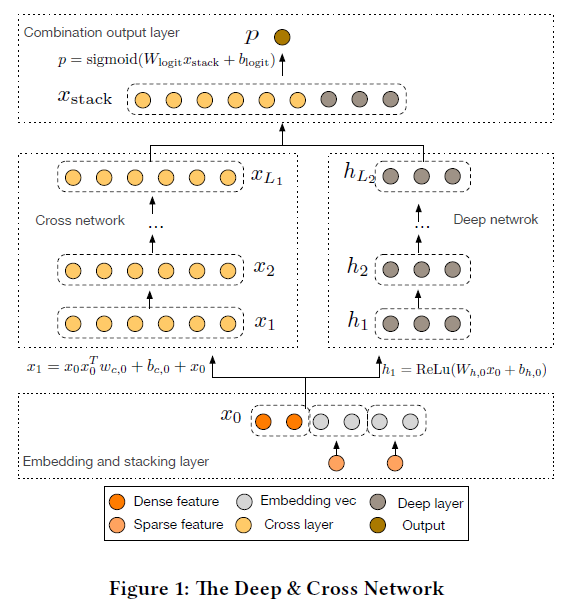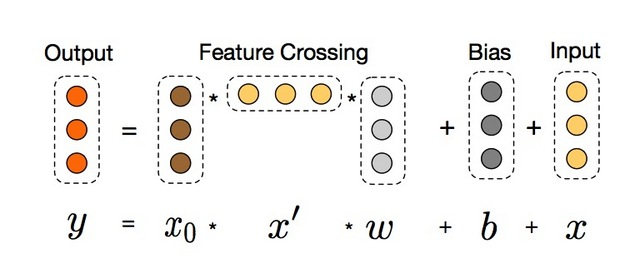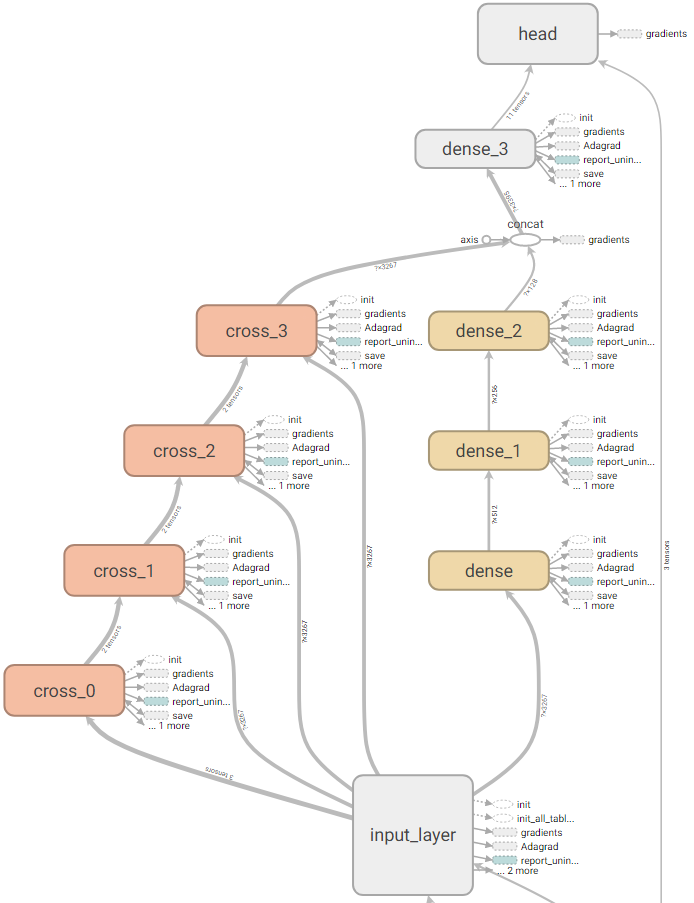# 小毛驴

Adventure may hurt you, but monotony will kill you.

0%

Deep & Cross Network(DCN)在 2017 年由 google 和 Stanford 共同发表的一篇论文中被提出，类似于Wide & Deep Network(WDL)，是用负杂网络预估CTR的一种方法。

## DCN网络结构

DCN模型以一个嵌入和堆叠层(embedding and stacking layer)开始，接着并列连一个cross network和一个deep network，接着通过一个combination layer将两个network的输出进行组合。## 交叉网络

• $x_l,x_{l+1}$是列向量（column vectors），分别表示来自第$l$层和第($l+1$)层cross layers的输出；
• $w_l, b_l \in R^d$是第$l$层layer的weight和bias参数。## 深度网络

• $h_l \in R^{n_l}, h_{l+1} \in R^{n_{l+1}}$别是第$l$层和第($l+1$)层hidden layer；
• $W_l \in R^{n_{l+1} \times n_l}, b_l \in R^{n_{l+1}}$第$l$个deep layer的参数；
• $f(⋅)$是ReLU function。

## Combination Layer## 总结

DCN主要有以下几点贡献：

• 提出一种新型的交叉网络结构，可以用来提取交叉组合特征，并不需要人为设计的特征工程；
• 这种网络结构足够简单同时也很有效，可以获得随网络层数增加而增加的多项式阶（polynomial degree）交叉特征；
• 十分节约内存（依赖于正确地实现），并且易于使用；
• 实验结果表明，DCN相比于其他模型有更出色的效果，与DNN模型相比，较少的参数却取得了较好的效果。

## 论文阅读

Deep & Cross Network for Ad Click Prediction